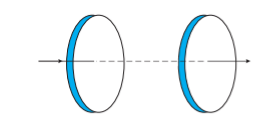Home/Class 12/Physics/

Figure shows a capacitor made of two circular plates each of radius $$12\;\text{cm}$$ , and separated by $$5.0\;\text{cm.}$$  The capacitor is being charged by an external source (not shown in the figure). The charging current is constant and equal to $$0.15\;A$$ .
Calculate the capacitance and the rate of charge of potential difference between the plates.Speed
00:00
04:04## QuestionPhysicsClass 12

Figure shows a capacitor made of two circular plates each of radius $$12\;\text{cm}$$ , and separated by $$5.0\;\text{cm.}$$  The capacitor is being charged by an external source (not shown in the figure). The charging current is constant and equal to $$0.15\;A$$ .
Calculate the capacitance and the rate of charge of potential difference between the plates.Distance between the capacitor plates, $$d=5\;\text{cm}=0.05m$$
Radius of circular plate, $$r=12\;\text{cm}=0.12\;m$$
Constant charging current, $$I=0.15A$$
Permittivity of free space,   $$\epsilon _o=8.85\times 10^{-12}C^2N^{-1}m^{-2}$$
Capacitance between the plates is given by
$$C=\dfrac{\mathit{\epsilon_o A}} d$$
Where,
$$A=$$  Area of each circular plate $$=\pi r^2.$$
$$C=\dfrac{\epsilon _o\pi r^2} d$$
= $$\dfrac{8.85\times 10^{-12}\times \pi \times (0.12)^2}{0.05}$$
$$=8.0032\times 10^{-12}F=80.032\mathit{pF}$$
Charge on each plate, $$q=\mathit{CV}$$
Where,
$$V$$  Potential difference across the plates
Differentiating it with respect to time $$(t)$$  gives:
$$\dfrac{\mathit{dq}}{\mathit{dt}}=C\dfrac{\mathit{dV}}{\mathit{dt}}$$
But, $$\dfrac{\mathit{dq}}{\mathit{dt}}$$  = current $$(I)$$
$$\dfrac{\mathit{dV}}{\mathit{dt}}$$  = $$\dfrac I C$$
$$\dfrac{0.15}{80.032\times 10^{-12}}$$ = $$1.87 × 10^9\;V / s$$
Therefore, the change in potential difference between the plates is $$1.87\times 10^9\ V/\mathit{s.}$$